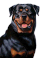cancel
Showing results for
Did you mean:Helper I

## Data modeling - Non unique values

Hello all!

First I'd like to thank all who read this and intend to help, you make this comunnity awesome!

I'm trying to link 2 tables, a FACT and a DIMENSIONS one, but this latter doesn't have unique values. Let me try to exemplify:So,  I would like to get something like this... If the ticket matches the 2 tables AND the 'DIM a'=10

The result should be this:Do anyone knows how to do that in DAX? Tried the VLOOKUP formula but didn't succeeded.

2 ACCEPTED SOLUTIONSCommunity Champion

@Tulio_DL

Hi, using a calculated column And DimA is 10

```When DimA-10 =
LOOKUPVALUE (
Table1[Dim B],
Table1[Ticket], Tickets[Ticket];
Table1[Dim A], 10
)```

Using a measure:

```Measure-When DimA-10 =
IF (
HASONEVALUE ( Table1[Ticket] ),
LOOKUPVALUE (
Table1[Dim B],
Table1[Ticket], VALUES ( Tickets[Ticket] ),
Table1[Dim A], 10
)
)```

let me know if works.

Victor

Lima - PeruMicrosoft

@Tulio_DL,

Create the calculated column in TABLE A.

```When DimA-10 =
LOOKUPVALUE (
'TABLE B'[DIM b],
'TABLE B'[Ticket], 'TABLE A'[Ticket],
'TABLE B'[DIM a], 10
)```Also you can change the second formula of Vvelarde to the following:

```Measure-When DimA-10 =
IF (
HASONEVALUE ( 'TABLE B'[Ticket] ),
LOOKUPVALUE (
'TABLE B'[DIM b],
'TABLE B'[Ticket], VALUES ( 'TABLE A'[Ticket] ),
'TABLE B'[DIM a], 10
)
)```

Regards,

Community Support Team _ Lydia Zhang
If this post helps, then please consider Accept it as the solution to help the other members find it more quickly.
8 REPLIES 8Community Champion

@Tulio_DL

Hi, using a calculated column And DimA is 10

```When DimA-10 =
LOOKUPVALUE (
Table1[Dim B],
Table1[Ticket], Tickets[Ticket];
Table1[Dim A], 10
)```

Using a measure:

```Measure-When DimA-10 =
IF (
HASONEVALUE ( Table1[Ticket] ),
LOOKUPVALUE (
Table1[Dim B],
Table1[Ticket], VALUES ( Tickets[Ticket] ),
Table1[Dim A], 10
)
)```

let me know if works.

Victor

Lima - PeruHelper I

@Vvelarde

Hello Victor!

I don't quite got there yet, could you please check your formula spelling? Let's say that the left table is named TABLE A (wich is where I have the facts) and the right one is TABLE B (wich there's the dimension I want to bring to TABLE A)Microsoft

@Tulio_DL,

Create the calculated column in TABLE A.

```When DimA-10 =
LOOKUPVALUE (
'TABLE B'[DIM b],
'TABLE B'[Ticket], 'TABLE A'[Ticket],
'TABLE B'[DIM a], 10
)```Also you can change the second formula of Vvelarde to the following:

```Measure-When DimA-10 =
IF (
HASONEVALUE ( 'TABLE B'[Ticket] ),
LOOKUPVALUE (
'TABLE B'[DIM b],
'TABLE B'[Ticket], VALUES ( 'TABLE A'[Ticket] ),
'TABLE B'[DIM a], 10
)
)```

Regards,

Community Support Team _ Lydia Zhang
If this post helps, then please consider Accept it as the solution to help the other members find it more quickly.Helper I

Got it working.. Thank you all

@Vvelarde

@v-yuezhe-msftAnonymous
Not applicable

I can no wrap my head around the way you are using dimension and fact tables.  I feel like you have them swapped.

Dimension tables should have unique id's...Helper I

Hello @Anonymous

It really looks like....... actually it is very confusing! BUT.... that's the only way a *** system gives me the information I need

='[Anonymous
Not applicable

Well, given you DO have a table of unique values, I would go ahead and create the relationship between ticket id's.  The 1 to many direction will be a bit opposite of what people think, but so be it.

Now, in your table of unique values, apparently you want a new calculated column... that looks up into the non-unique table, and does *what* exactly?Helper I

Yes @Anonymous! I have already established this relationship, and yes it's totally in the opposite way...

I would like to bring the information at 'DIMENSION table'[DIM b] into my 'FACT table'

considering that 'DIMENSION table'[DIM a] = 10     for exampleAnnouncements#### Exclusive opportunity for Women!

Join us for a free, hands-on Microsoft workshop led by women trainers for women where you will learn how to build a Dashboard in a Day!#### Power Platform Conference-Power BI and Fabric Sessions

Join us Oct 1 - 6 in Las Vegas for the Microsoft Power Platform Conference.Top Solution Authors
Top Kudoed Authors
Users online (2,713)